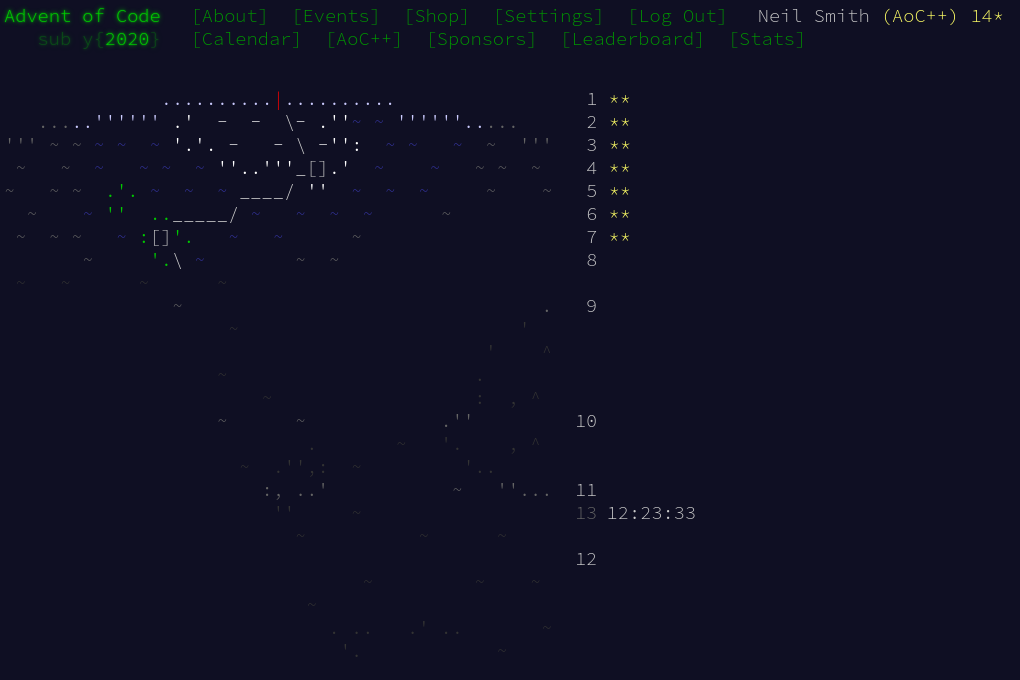December 14, 2020

# Advent of Code 2020 day 7

Bags and DAGsDay 7 was unusual in that I found part 1 harder than part 2. Also, the data structures in today's solution are a mess. (But see the addendum below.)

Fundamentally, today was about walking across a directed acyclic graph. (At least, I assumed the input was acyclic: I didn't check, and things could have gone very wrong if there were cycles.)

## Parsing

This was another outing for Attoparsec, with nothing particularly special. The end result is a Map of bag names to the set of bags it contains. (There are also a couple of declarations needed for part 1.)

data QuantifiedBag = QuantifiedBag Integer String
deriving (Show, Eq, Ord)

qName (QuantifiedBag _ n) = n
qCount (QuantifiedBag n _) = n

type Bags = S.Set String
type QBags = S.Set QuantifiedBag
type BagRules = M.Map String QBags
type InvertBags = M.Map String Bags


A bag name is a bunch of characters, up to but not including " bag" or " bags". The contents of a bag are either empty or a list of bags separated by commas.

bagNameP = manyTill anyChar ((string " bags") <|> (string " bag"))

quantifiedBagP = QuantifiedBag <$> decimal <* space <*> bagNameP emptyBagListP = "no other bags" *> pure S.empty bagListP = S.fromList <$> sepBy quantifiedBagP (string ", ")

bagContentsP = emptyBagListP <|> bagListP

ruleP = (,) <$> bagNameP <* " contain " <*> bagContentsP <* "." rulesP = M.fromList <$> sepBy ruleP endOfLine


A couple of things to note:

• the the use of pure to return a particular value when there's an empty bag list
• the inclusion of full stops at the end of each line means the parser can treat each line individually (rather than wondering if there's an extra 's' after finding 'bag')

## Part 1

Given a graph of bags of the form "this bag contains that bag", I had to find all the "this bags" for a given "that bag", and the transitive closure of that relation. Given I'm storing the rules in a Map, that involves finding the keys for a particular value. Rather than searching through the whole Map every time, I decided to pre-process the rules and invert them. That would give me a Map with rules of the form "this bag is contained in that bag". From there, finding all the containing bags would be fairly easy.

Because one bag could be contained in many others, I had to break apart the rules into their atomic 1:1 connections, invert them, and use that to rebuild the inverted bag Map.

invertBags :: BagRules -> InvertBags
invertBags bags = foldr addInvert M.empty $concatMap swapPair$ M.assocs bags
where swapPair (a, bs) = [(qName b, a) | b <- S.toList bs]

addInvert :: (String, String) -> InvertBags -> InvertBags
addInvert (k, v) m = M.insert k v' m
where v' = S.insert v (M.findWithDefault S.empty k m)


From there, it's an exhaustive search across that graph, in something like a breadth-first manner. The agenda is the bags to find containers for, the results are the bags found already. At each step, remove a bag from the agenda, add it to the results. If it's not already in the results, look up the containing bags and add them to the agenda.

where others = S.toList $bags!thisBag subCount b = (qCount b) * (nContainedBags bags (qName b))  ## Addendum: using a graph library The structure of bag containments is a graph, so it would make sense to take advantage of an existing library for handling graphs. Hackage includes several graph libraries, such containers, fgl, graphs, graph-wrapper, and graphite. Most of them, such as the graphs in containers, are restricted in the data they can store in the graph, often labelling vertexes as Int and having edges without weights. Graphite, however, does allow arbitrary values for vertexes and labelled edges. It also has some very good, comprehensive documentation. The library doesn't do much reexporting, so there are more imports that you'd think. A BagGraph has vertexes of type String (the bag name) and arcs annotated with type Int (the number of bags). To build a graph from the rules, I add all the arcs for each rule in turn; to add the arcs from a rule, I add the arcs for each contained bag in turn; to add an arc, I just insertArc it. The vertexes are automatically created in the graph as needed. import qualified Data.Graph.DGraph as D import qualified Data.Graph.Types as G import qualified Data.Graph.Traversal as T type BagGraph = D.DGraph String Int buildGraph :: BagRules -> BagGraph buildGraph rules = M.foldrWithKey addRule G.empty rules addRule :: String -> QBags -> BagGraph -> BagGraph addRule source dests graph = S.foldr (addArc source) graph dests addArc :: String -> QuantifiedBag -> BagGraph -> BagGraph addArc source (QuantifiedBag quantity destination) graph = D.insertArc arc graph where arc = G.Arc source destination quantity  Part 1 is now just a case of transposing the graph (reversing the direction of each arc) and finding the number of vertexes from the god bag. Part 2 is an exhaustive graph search, where the results of a sub-search are multiplied by the edge weight. part1 graph = length (T.bfsVertices (D.transpose graph) "shiny gold") - 1 part2 graph = (bfsCount graph "shiny gold") - 1 bfsCount :: BagGraph -> String -> Int bfsCount graph thisBag = 1 + (sum$ map subCount others)
where others = D.outboundingArcs graph thisBag
subCount a = (G.attribute a) * (bfsCount graph \$ G.destinationVertex a)


## Code

You can find the code here or on Gitlab.